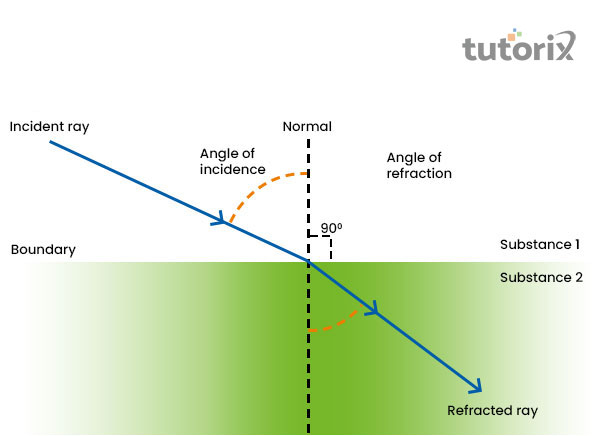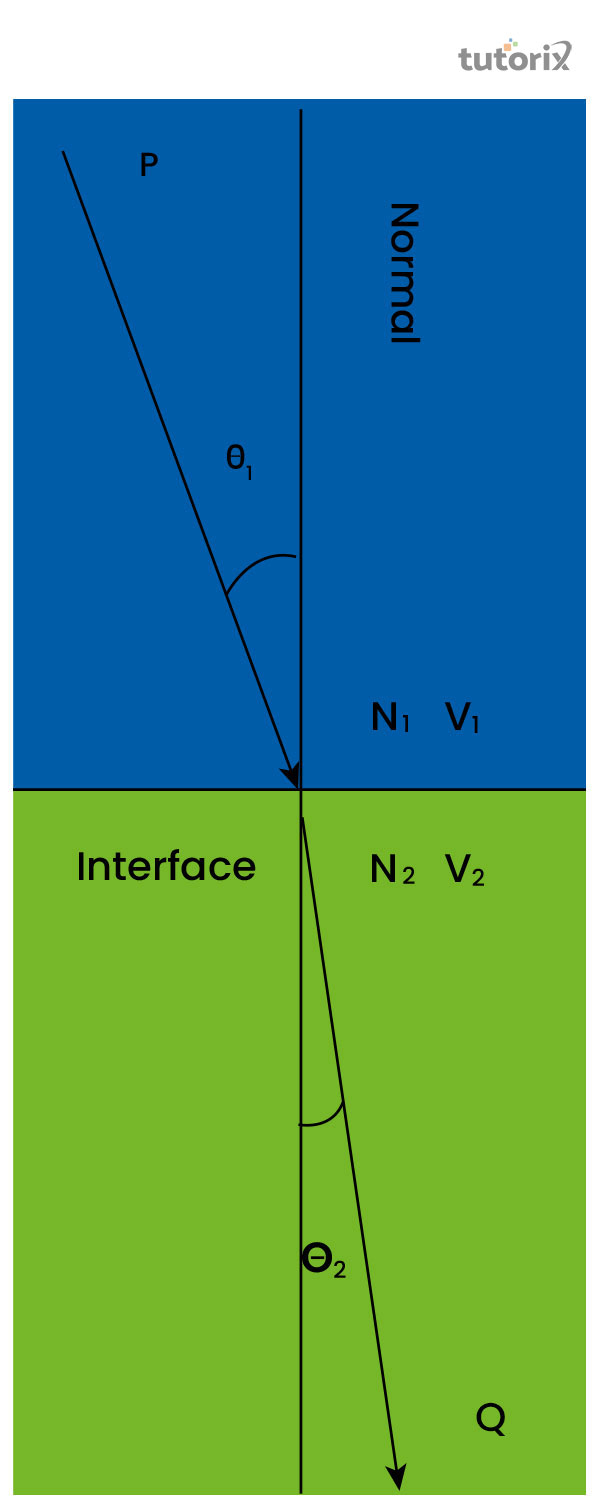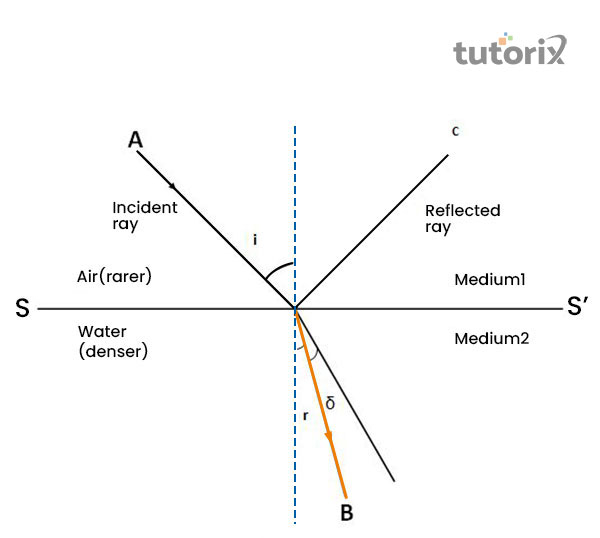# Refraction of Light

## Introduction

Light travels a straight path when it bends while travelling in a single direction. A certain change in the speed of light causes a directional change in its path and that is when refraction happens after light passes a boundary from one transparent medium to another. The bending of light by refraction makes it possible for the human retina to observe prisms and rainbows. Without refraction of light, the human eye cannot focus on the pathway of light.

## What is Refraction of Light

Refraction refers to the process of bending light immediately after it passes from one transparent substance to another substance. It happens with other waves like sound and water and the process of refraction is solely responsible for enabling the human eye to have lenses, prisms and rainbows. Refraction also allows the human eye to focus and without the refraction of light, one cannot focus on substances (Sciencelearn, 2022).Figure 1: Refraction of light

Refraction of light mostly occurs when a wave of light is at angle away through its normal path and then passes the boundary from one medium to second one.

Here, a change of velocity takes place in the pathway of light which is mostly controlled by the process of refraction. While travelling from one angle to a different medium, the process of refraction follows a different refractive index (Malik et al. 2021). After the deviation of the part of light takes place, a ray of light can be seen refracting according to its nature.

## Types of Refraction

### From denser to rarer medium

In refraction from the denser medium to a rarer medium, the refractive index appears less than 1 and the angle of incidence appears less than the angle of refraction. Here, the refracted ray becomes 10 in order to shift away from the normal medium (Leverageedu, 2022). Between glass and air, when the refraction of air takes place, it travels from a denser medium to a rarer medium.

### From rarer to denser medium

In case of refraction from a rarer medium to a denser medium, the reactive reflective index becomes greater than 1 and the angle of incidence appears greater than the angle of refraction. After that, the refracted ray intends to shift towards the normal medium (Leverageedu, 2022). When refraction of light happens between air and water the light travellers from a rarer medium to a denser medium.

### Laws of Refraction of Light

The laws of refraction of the light state that the incident ray, the refracted ray and the normal to the interface of two media at the point of incidence lie on the same plane. Apart from this refraction of light also follows the ratio of the sine of the angle of refraction which appears as constant during the process of refraction (Dido et al. 2021). This law is also known as the law of refraction by Snell.

### Snell's law

Snell's Law of refraction is an essential component for understanding the way light is refracted while travelling from one medium to another. Snell's law talks about the angle of the bending ray on the indices of refraction of the two mediums of light.Figure 2: Snell's Law

Snell's law is derived from the Fresnel Equations or Fermat's Principle where it states that the ratio of the sine in the angle of incidence is constant to the sine of the angle of refraction.

The formula of Snell's Law of refraction is n1/n2 = sin θ2/ sin θ1 (Leverageedu, 2022).

## Causes of Refraction of Light

Refraction of light generally takes place when the frequency of the refracted ray remains the same for a longer time. When the intensity of the refracted rays appears less than an incident ray, the process of refraction of light takes place automatically. It only happens when absorption of light and partial reflection touches the interface of a medium (Sciencelearn, 2022).Figure 3: Causes of refraction

Apart from that, refraction also happens when the light crosses the boundary and the process of deviation takes place. In addition, it happens when the wavelength of light and the speed of light vary (Dido et al. 2021).

The process of refraction generally takes place between different media where light travels from a transparent medium to another medium.

## Conclusion

Refraction of light is an essential process that allows the human retina to see the pathway of light. This process is mostly triggered by the stagnant frequency of the refracted rays and a higher intensity of the incident rays. For example, when a white light is passed through a prism, it gets split into seven different colour components, due to the refraction of light.

## FAQs

Q1. What are some of the applications of reflection of light?

Refraction is related to optics technology, so whenever a lens is used for forming a magnifying image, the process of refraction takes place. It is also applied to the twinkling of stars and mirage.

Q2. What is the refractive index?

Refractive index is the way of measuring the bending of light after it passes from one transparent medium to another. It is denoted as n = c/v where the velocity of light ray has a ratio with the velocity of light substance.

Q3. What is the refractive index of transparent substances like air, water and diamond?

The refractive index of air is 1.00 and for water, the index is 1.33. Similarly, for diamonds, the refractive index is 2.4, the highest of all transparent substances.

Q4. What are some real-life examples of refraction of light?

The formation of a rainbow and a mirage is the most appropriate example of refraction of light. Another example is when a swimming pool looks shallow; it is actually its depth that is caused by the refraction of light.

## References

### Book

Fortson, N. (2022). Discovering the Nature of Light: The Science and the Story. Retrieved from: https://www.worldscientific.com

### Journals

Dido, F., Mongan, S., Mandang, T., Palilingan, R., Mondolang, A., & Poluakan, C. (2021, July). Analysis of student difficulties in learning refraction of light. In Journal of Physics: Conference Series (Vol. 1968, No. 1, p. 012033). IOP Publishing. Retrieved from: https://iopscience.iop.org

Malik, A., Mardianti, D., Nurlutfiah, D., Izzah, D. W., Mulhayatiah, D., Nasrudin, D., & Suhendi, H. Y. (2021, March). Determination of refractive index on three mediums based on the principle of refraction of light. In Journal of Physics: Conference Series (Vol. 1806, No. 1, p. 012003). IOP Publishing. Retrieved from: https://iopscience.iop.org

## Websites

Leverageedu, (2022). Refraction of light. Retrieved from: https://leverageedu.com [Retrieved on 9th June, 2022]

Sciencelearn, (2022).Causes of refraction of light. Retrieved from: https://www.sciencelearn.org.nz [Retrieved on 9th June, 2022]